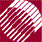ECE532 Biomedical Optics © 1998 Steven L. Jacques, Scott A. Prahl Oregon Graduate Institute

# Diffusion theory

The time-resolved fluence rate F(r,t) [W/cm2] in response to an impulse of energy Uo [J] and the steady-state fluence rate Fss(r) [W/cm2] in response to an isotropic point source of continuous power Po [W] are summarized: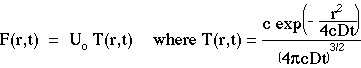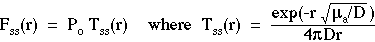where the transport factors T(r,t) [cm-2 s-1] and Tss(r) [cm-2] have been introduced to more carefully distinguish the source, the transport, and the fluence rate.

Note on notation: In this class, we use Fss(r) rather than F(r) to especially emphasize the steady-state fluence rate from the time-resolved fluence rate. However, F(r) should be used outside this class.

The above expression for Tss(r) can be obtained by integrating T(r,t)exp(-µact) over all time to yield the total accumulated amount of photon transport to each position r. The factor exp(-µact) accounts for photon absorption (recall that ct = pathlength so this expression is simply Beer's law for photon survival) and causes photon concentration to approach zero as time goes to infinity. The expression for Tss(r) is derived: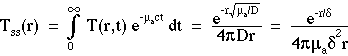Note that the final expression above has made the substitutions:which removes the diffusion length D and introduces the optical penetration depth δ which is the incremental distance from the source that causes Fss(r) to decrease to 1/e its initial value. The penetration depth δ is a parameter which is very easily understood in experimental measurements and consequently has more intuitive value to some people than D which is important from the perspective of the local step size of the diffusion process. In this class we will often use the following expression for Fss(r) when we prefer to emphasize the roles of µa and δ: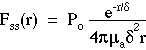to next page | Chapter 5 | Course | Home